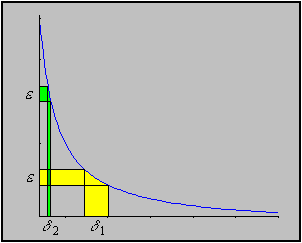#Interactive Real Analysis

Next | Previous | Glossary | Map

## 6.2. Continuous Functions

### Example 6.2.8(a):

The function f(x) = 1 / x is continuous on (0, 1). Is it uniformly continuous there ?
It helps to look at the graph of the function:As the- interval 'slides' up the positive y-axis, the corresponding-interval on the x-axis gets smaller and smaller. That indicates that the function is not uniformly continuous - but it is of course not a proof. So:

Take any= 1. Does there exist anysuch that

if | t - s | <then | f(t) - f(s) | <= 1 ?

The basic idea is easy: since | f(t) - f(s) | = | 1/t - 1/s | = | s - t | * 1 / | st |, we can see that this might approach infinity if s and t approach zero, and therefore will be bigger than any chosen. All we have to do now is formalize this proof.

Assume there exists such a. Without loss of generality we may assume that< 1 (why ?). Then let s = t +/ 2 and set t =/ 2. We have (assuming that s, t are positive):

| f(t) - f(s) | = | 1/s - 1/t | = | (t - s) / st | => 1

But now, no matter what< 1 is we can make | f(t) - f(s) | > 1. Therefore, the function is not uniformly continuous.

This proof, loosely speaking, depends on the fact that after simplification | f(t) - f(s) | goes to infinity if s and t approach zero. That is exactly the situation as described in the above picture.

Next | Previous | Glossary | Map